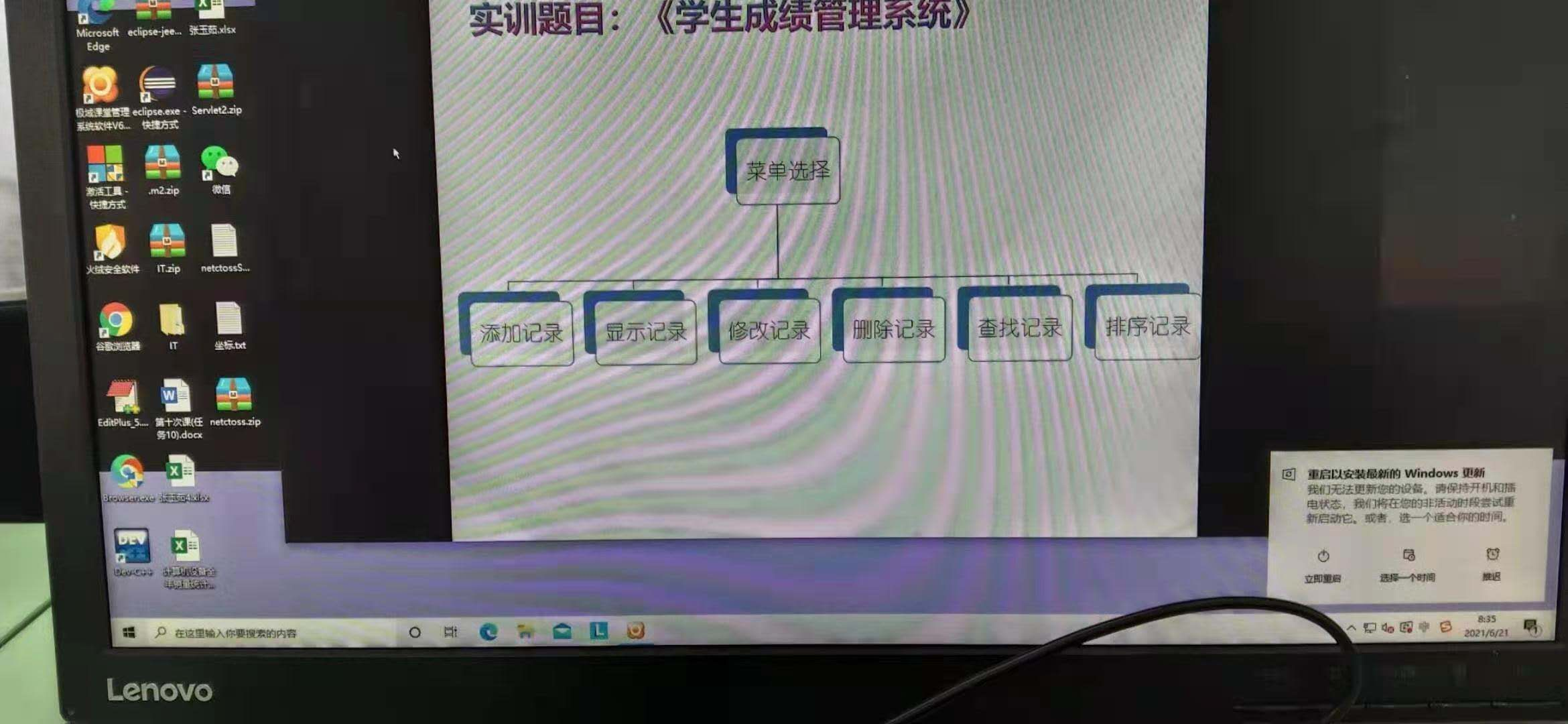2021-06-21 14:58

# 学生成绩管理系统程序代码• 写回答
• 关注问题
• 收藏
• 邀请回答

#### 1条回答默认 最新

•CSDN专家-Fay 2021-06-21 15:09
已采纳

类似代码拿过去修改一下：

``````/* Note:Your choice is C IDE */
#include "stdio.h"
struct Student{
int stuno;//学号
char stuname;//姓名
char sex;//性别
char classname;//班级名称
int yy;//英语课成绩
int sx;//数学课成绩
int yw;//语文课成绩
}stu;
int i=0;//记录当前用户数
//1、信息录入
void xxlr(){
printf("********学生信息录入*******\n");
printf("请输入学号：");
scanf("%d",&stu[i].stuno);

printf("请输入学生姓名：");
scanf("%s",&stu[i].stuname);

printf("请输入性别（男/女）：");
scanf("%s",&stu[i].sex);

printf("请输入班级：");
scanf("%s",&stu[i].classname);

printf("请输入该学生英语，数学，语文三门成绩，用空格隔开，回车结束:");
scanf("%d %d %d",&stu[i].yy,&stu[i].sx,&stu[i].yw);
i++;//用户数加1
printf("\n录入成功，按回车回到主界面：");
getch();//暂停
system("CLS");//清屏
}
//2、信息显示
void xs(){
int n;
printf("********显示学生信息*******\n\n");
printf("\t学号\t姓名\t性别\t班级\t英语\t数学\t语文\n\n");
for(n=0;n<i;n++){
printf("\t%d\t%s\t%s\t%s\t%d\t%d\t%d\n\n",stu[n].stuno,stu[n].stuname,stu[n].sex,stu[n].classname,stu[n].yy,stu[n].sx,stu[n].yw);
}
printf("\n按回车回到主界面：");
getch();//暂停
system("CLS");//清屏
}
//3、按姓名查找
void cz(){
char sname;
int n;
int flag=0;//没找到
printf("********查找学生信息*******\n\n");
printf("请输入要查找的学生姓名：");
scanf("%s",&sname);
for(n=0;n<i;n++){
if( strcmp(stu[n].stuname,sname)==0){
printf("\t学号\t姓名\t性别\t班级\t英语\t数学\t语文\n\n");
printf("\t%d\t%s\t%s\t%s\t%d\t%d\t%d\n\n",stu[n].stuno,stu[n].stuname,stu[n].sex,stu[n].classname,stu[n].yy,stu[n].sx,stu[n].yw);
flag=1;
break;
}
}
if(flag==0){
printf("没有找到相关学生\n");
}
printf("按回车退出:");
getch();//暂停
system("CLS");//清屏
}
//4、按性别显示每门课程的平均分
void pjf(){
int n,na=0,nv=0;//na男生个数 nv女生个数
float yy1=0,sx1=0,yw1=0;//男生三门课总分数
float yy2=0,sx2=0,yw2=0;//女生三门课总分数
printf("********查询每门课程平均分（按性别）*******\n\n");
for(n=0;n<i;n++){
if(strcmp(stu[n].sex,"男")==0){
yy1+=stu[n].yy;
sx1+=stu[n].sx;
yw1+=stu[n].yw;
na++;
}else if(strcmp(stu[n].sex,"女")==0){
yy2+=stu[n].yy;
sx2+=stu[n].sx;
yw2+=stu[n].yw;
nv++;
}
}
printf("\t性别\t英语\t数学\t语文\n\n");
if(na>0){
printf("\t男\t%.2f\t%.2f\t%.2f\n\n",yy1/na,sx1/na,yw1/na);
}
if(nv>0){
printf("\t女\t%.2f\t%.2f\t%.2f\n\n",yy2/nv,sx2/nv,yw2/nv);
}
printf("按回车退出:");
getch();//暂停
system("CLS");//清屏
}
//5、每门课程最高和最低
void zgd(){
int n;
int zg1=0,zg2=0,zg3=0;
int zd1=10000,zd2=10000,zd3=1000;
printf("********查询每门课程最高和最低分*******\n\n");

for(n=0;n<i;n++){
//英语最高和最低
if(stu[n].yy>zg1){
zg1=stu[n].yy;
}
if(stu[n].yy<zd1){
zd1=stu[n].yy;
}

//数学最高和最低
if(stu[n].sx>zg2){
zg2=stu[n].sx;
}
if(stu[n].sx<zd2){
zd2=stu[n].sx;
}

//语文最高和最低
if(stu[n].yw>zg3){
zg3=stu[n].yw;
}
if(stu[n].yw<zd3){
zd3=stu[n].yw;
}

}
printf("\t类别\t英语\t数学\t语文\n\n");
printf("\t最高\t%d\t%d\t%d\n\n",zg1,zg2,zg3);
printf("\t最低\t%d\t%d\t%d\n\n",zd1,zd2,zd3);

printf("按回车退出:");
getch();//暂停
system("CLS");//清屏
}
//6、删除学生信息
void sc(){
int n;
int xh;
int flag=0;//没找到
char ss;

int k;
printf("********删除学生信息*******\n\n");
printf("请输入要删除的学生学号：");
scanf("%d",&xh);
for(n=0;n<i;n++){
if(stu[n].stuno==xh){
flag=1;
break;
}
}
if(flag==0){
printf("没有找到该学生!\n");
}else{
printf("\t学号\t姓名\t性别\t班级\t英语\t数学\t语文\n\n");
printf("\t%d\t%s\t%s\t%s\t%d\t%d\t%d\n\n",stu[n].stuno,stu[n].stuname,stu[n].sex,stu[n].classname,stu[n].yy,stu[n].sx,stu[n].yw);
printf("确定要删除(y/n):");
fflush(stdin);
scanf("%c",&ss);

if(ss=='y'){
for(k=n;k<i;k++){
stu[k]=stu[k+1];
}
i--;//人数减一
printf("删除成功!!\n");
}
}

printf("按回车退出:");
getch();//暂停
system("CLS");//清屏

}
void main()
{

int gn;//接收用户功能输入

printf("\t\t****************************************\n");
printf("\t\t*                                      *\n");
printf("\t\t*                                      *\n");
printf("\t\t*         班级成绩管理系统             *\n");
printf("\t\t*                                      *\n");
printf("\t\t*                                      *\n");
printf("\t\t****************************************\n");
do{
printf("\n");
printf("\t\t*        1、学生信息录入               *\n");
printf("\t\t*        2、显示所有学生信息           *\n");
printf("\t\t*        3、按姓名查找学生             *\n");
printf("\t\t*        4、查询课程平均成绩（按性别） *\n");
printf("\t\t*        5、课程最高和最低成绩查询     *\n");
printf("\t\t*        6、删除学生信息               *\n");
printf("\t\t*        7、退出系统                   *\n");
printf("\n\t\t请选择你要操作的功能：");
scanf("%d",&gn);
printf("\n");
if(gn==1){
xxlr();//调用信息录入函数
}else if(gn==2){
xs();
}else if(gn==3){
cz();
}else if(gn==4){
pjf();
}else if(gn==5){
zgd();
}else if(gn==6){
sc();
}

}while(gn!=7);
}``````
已采纳该答案
打赏 评论